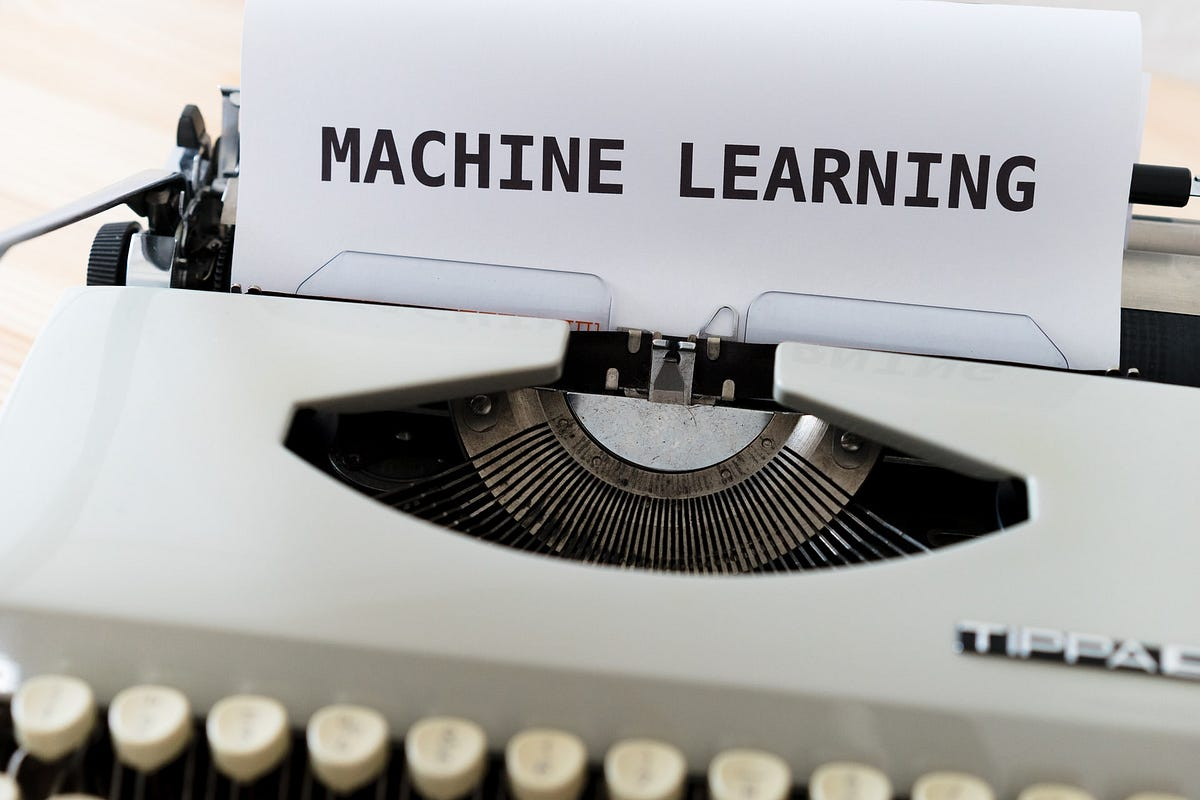# Logistic Regression in Classification model using Python: Machine LearningLearning how to build a basic logistic regression model in machine learning using python . Logistic regression is a commonly used model in various industries such as banking, healthcare because when compared to other classification models, the logistic regression model is easily interpreted.

Logistic regression is a commonly used model in various industries such as banking, healthcare because when compared to other classification models, the logistic regression model is easily interpreted.

### Binary Classification

Binary classification is the most commonly used logistic regression. Some of the examples of binary classification problems are:

• A finance company wants to know whether a customer is default or not
• Predicting an email is spam or not
• Whether a person is diabetic or not

The binary classification always has only two possible outcomes, either ‘yes’ & ‘no’ or ‘1’ & ‘0’ etc.

Like in the previous article “Multiple Linear Regression model, “ one independent variable is often not enough to capture all the uncertainties of the logistic regression’s target variable.

## Hire Machine Learning Developers in India

We supply you with world class machine learning experts / ML Developers with years of domain experience who can add more value to your business.

## Linear Regression VS Logistic Regression (MACHINE LEARNING)

Linear Regression VS Logistic Regression (MACHINE LEARNING). Linear Regression and Logistic Regression are two algorithms of machine learning and these are mostly used in the data science field.

## Python "Scikit-Learn": Logistic Regression Classification

Learn how to apply logistic regression for binary classification by making use of the scikit-learn package within Python

## Logistic Regression for Machine Learning using Python

Logistic Regression for Machine Learning using Python. Logistic regression is easy to interpretable of all classification models. It is very common to use various industries such as banking, healthcare, etc.

## Classification In Machine Learning | Machine Learning Tutorial | Python Training

Machine Learning can perform a lot of tasks, such as regression, classification, clustering, etc. Learn about Classification in Machine Learning. You will know what classification is and some of its essential terminologies. You will understand the various applications and look into some of the vital classification algorithms in Machine Learning. You will learn about Logistics Regression, KNN, SVM, Decision Trees, and perform a hands-on demo for each in Python.